Geometry / Mr. Hansen 3/4/2003 [fonts corrected 2/16/2009] Name: _______________________

Angle-Arc Puzzles Created by Students

 1. Frank F.Given: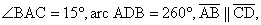tangents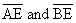Find: All other angles and arcs 2. Robin K.Given: Circle R, arc BF = 50°, tangents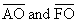(1) Without computing the measure of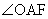, determine if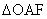is right. (2) Now computeand all other angles and arcs. 3. Michael L. (edited by Mr. Hansen, since original version had infinitely many solutions)Given: Circle P, arc CF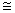arc FB,= 68°, arc BD = 74°, tangent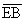Find: All other angles and arcs 4. Alex W.Given: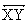bisects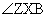, arc WZQ = 100°,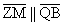Find: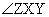and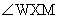5. Sam W.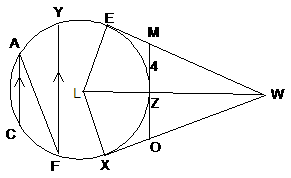Given: Circle L with tangents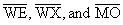,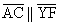,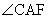= 10°,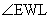= 30°, MZ = 4 as marked Find: every angle, arc, and segment length (including radius of circle L) that can be determined